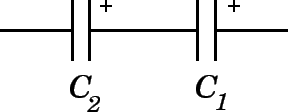# A 330 pF capacitor and a 220 pF capacitor in series are each connected across a 6 V dc source. What is the voltage across the 330 pF capacitor?

Sep 27, 2015

$2.4 \text{V}$

#### Explanation:For capacitors in series like this the total charge stored by each capacitor is the same.

The capacitance $C$, charge stored $Q$ and the voltage $V$ are related by:

$C = \frac{Q}{V}$

So:

$Q = C V$

Let ${C}_{1} = 330 \text{pF}$

and ${C}_{2} = 220 \text{pF}$

So:

${C}_{1} {V}_{1} = {C}_{2} {V}_{2}$ $\text{ } \textcolor{red}{\left(1\right)}$

The voltage drop across the 2 capacitors = 6V so we can write:

${V}_{1} + {V}_{2} = 6$ $\text{ } \textcolor{red}{\left(2\right)}$

We have 2 simultaneous equations here so from $\textcolor{red}{\left(2\right)}$ we can write:

${V}_{2} = \left(6 - {V}_{1}\right)$

We can now substitute this expression for ${V}_{2}$ into $\textcolor{red}{\left(1\right)} \Rightarrow$

${C}_{1} {V}_{1} = {C}_{2} \left(6 - {V}_{1}\right)$

${C}_{1} {V}_{1} = 6 {C}_{2} - {C}_{2} {V}_{1}$

${C}_{1} {V}_{1} + {C}_{2} {V}_{1} = 6 {C}_{2}$

${V}_{1} \left({C}_{1} + {C}_{2}\right) = 6 {C}_{2}$

${V}_{1} = \frac{6 {C}_{2}}{\left({C}_{1} + {C}_{2}\right)}$

${V}_{1} = \frac{6 \times 220 \times \cancel{{10}^{- 12}}}{\left(330 + 220\right) \times \cancel{{10}^{- 12}}}$

${V}_{1} = \frac{1320}{550} = 2.4 \text{V}$

• which is the voltage drop across the $330 \text{pF}$ capacitor.

This also means that ${V}_{2} = 6 - 2.4 = 3.6 \text{V}$